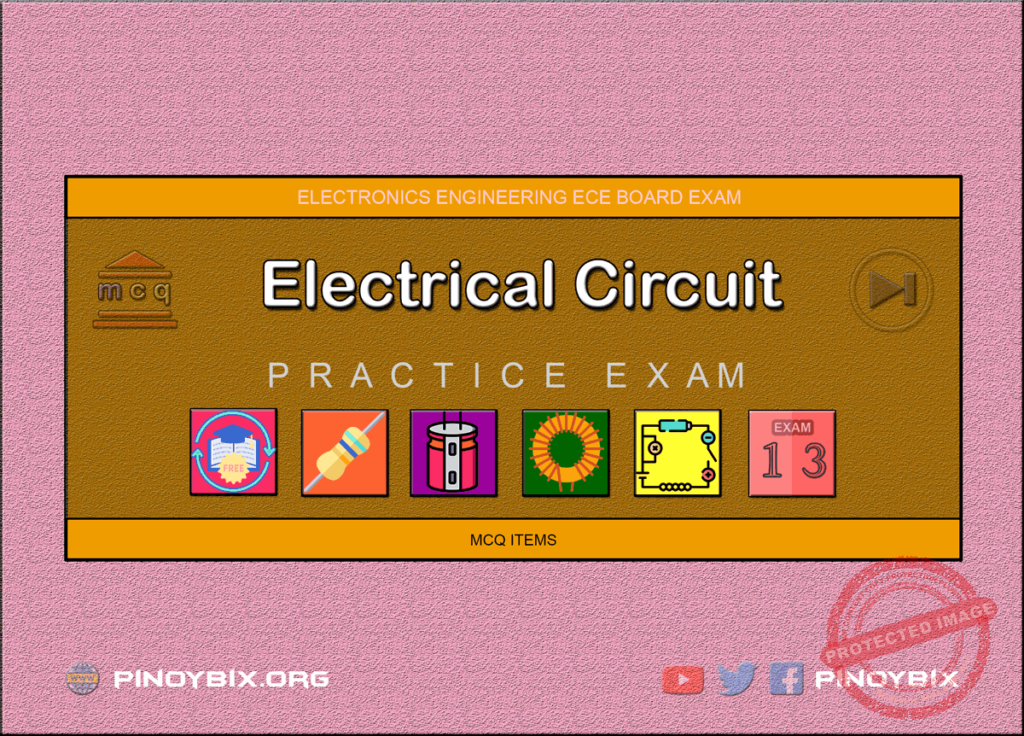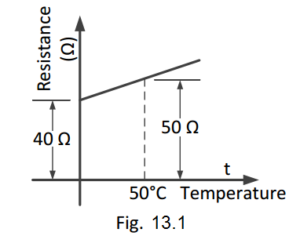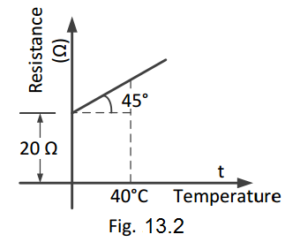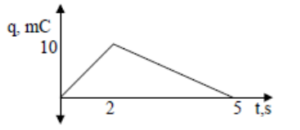# MCQ in Electrical Circuit Part 13 | ECE Board Exam

(Last Updated On: November 24, 2020)This is the Multiples Choice Questions Part 13 of the Series in Electrical Circuit as one of the Electronics Engineering topic. In Preparation for the ECE Board Exam make sure to expose yourself and familiarize in each and every questions compiled here taken from various sources including but not limited to past Board Exam Questions in Electronics Engineering field, Electronics Books, Journals and other Electronics References.

#### MCQ Topic Outline included in ECE Board Exam Syllabi

• MCQ in AC-DC circuits
• MCQ in Resistors
• MCQ in Inductors
• MCQ in Capacitors

#### Continue Practice Exam Test Questions Part 13 of the Series

Choose the letter of the best answer in each questions.

601. A certain wire 20 ft long and 100 circular mil area has a resistance of 1.6 Ω. What is its resistivity?

A. 10.3 ohm-CM/ft

B. 2.2 ohm-CM/ft

C. 8 ohm-CM/ft

D. 15.2 ohm-CM/ft

Solution:

602. How many circular mils does a round copper rod of 0.25 inch diameter have?

A. 196,000

B. 62,500

C. 1,963,500

D. 49,000

Solution:

603. A substance whose molecules consist of the same kind of atoms is called _________.

A. mixture

B. element

C. compound

D. none of these

Solution:

604. The diameter of the atom is about ___________.

A. 10^-10 m

B. 10^-8 m

C. 10^-2 m

D. 10^-15 m

Solution:

605. The number of compounds available in nature is ___________.

A. 105

B. 300

C. 1000

D. unlimited

Solution:

606. The mass of a proton is ___________ the mass of an electron.

A. equal to

B. less than

D. 200 times

Solution:

607. The maximum number of electrons that can be accommodated in the last orbit is _________.

A. 4

B. 8

C. 18

D. 2

Solution:

608. The electrons in the last orbit of an atom are called _________ electrons.

A. free

B. bound

C. valence

D. thermionic

Solution:

609. If the number of valence electrons of an atom is less than 4, the substance is usually __________.

A. a conductor

B. an insulator

C. a semiconductor

D. none of these

Solution:

610. If the number of valence electrons of an atom is more than 4, the substance is usually __________.

A. a semiconductor

B. a conductor

C. an insulator

D. none of these

Solution:

611. If the number of valence electrons of an atom is exactly 4, the substance is usually _________.

A. a semiconductor

B. an insulator

C. a conductor

D. a semiconductor

Solution:

612. The number of valence electrons of an atom is less than 4. The substance will be probably __________.

A. a metal

B. a non-metal

C. an insulator

D. a semiconductor

Solution:

613. One coulomb of charge is equal to the charge on __________ electrons.

A. 628 x 10^16

B. 6.28 x 10^16

C. 62.8 x 10^16

D. 0.628 x 10^16

Solution:

614. One cc of copper has about ___________ free electrons at room temperature.

A. 200

B. 20 x 10^10

C. 8.5 x 10^22

D. 3 x 10^5

Solution:

615. Electric current in a wire is the flow of _________.

A. free electrons

B. bound electrons

C. valence electrons

D. atoms

Solution:

616. EMF in a circuit is _________.

A. cause current to flow

B. maintains potential difference

C. increases the circuit resistance

D. none of these

Solution:

617. EMF has the unit of _________.

A. power

B. energy

C. charge

D. none of these

Solution:

618. Potential difference has the unit of _________ .

A. charge

B. power

C. energy

D. none of these

Solution:

619. The resistance of a material is ________ its area of cross-section.

A. directly proportional to

B. inversely proportional to

C. independent of

D. none of these

Solution:

620. If the length and area of cross-section of a wire are doubled up, then its resistance __________.

A. becomes four times

B. remains unchanged

C. becomes sixteen times

D. none of these

Solution:

621. A length of wire has a resistance of 6 ohms. The resistance of a wire of the same material three times as long and twice the cross-sectional area will be ________.

A. 36 ohms

B. 12 ohms

C. 9 ohms

D. 1 ohm

Solution:

622. The SI unit of specific resistance is _________.

A. mho

B. ohm-m

C. ohm-m^2

D. ohm-cm

Solution:

623. The specific resistance of a conductor _________ with rise in temperature.

A. increases

B. decreases

C. remains unchanged

D. none of these

Solution:

624. The SI unit of conductivity is ________.

A. ohm-m

B. ohm/m

C. mho-m

D. mho/m

Solution:

625. The SI unit of conductance is _______.

A. mho

B. ohm

C. ohm-m

D. ohm-cm

Solution:

626. The resistance of a material 2 m long and 2 m^2 in cross-sectional area is 1.6 x 10^-8 Ω. Its specific resistance will be __________.

A. 3.2 x 10^-8 ohm-m

B. 6.4 x 10^-8 ohm-m

C. 1.6 x 10^-8 ohm-m

D. 0.16 x 10^-8 ohm-m

Solution:

627. Conductors have ________ temperature coefficient of resistance.

A. positive

B. negative

C. zero

D. none of these

Solution:

628. Semiconductors have ________ temperature coefficient of resistance.

A. negative

B. positive

C. zero

D. none of these

Solution:

629. The value of α (i.e. temperature coefficient of resistance) depends upon

A. length of the material

B. cross-sectional area of the material

C. volume of the material

D. nature of the material and temperature

Solution:

630. The temperature coefficient of resistance of a conductor ________ with rise in temperature.

A. increases

B. decreases

C. remains unchanged

D. none of these

Solution:

631. Insulators have _________ temperature coefficient of resistance.

A. zero

B. positive

C. negative

D. none of these

Solution:

632. Eureka has _________ temperature coefficient of resistance.

A. positive

B. negative

C. almost zero

D. none of these

Solution:

633. Fig. 13.1 shows the temperature/resistance graph of a conductor. The value of α0 is ________.A. 0.005/°C

B. 0.004/°C

C. 0.1/°C

D. 0.4/°C

Solution:

634. Referring to Fig. 13.1, the value of the α50 will be ________.A. 0.005/°C

B. 0.004/°C

C. 0.1/°C

D. 0.4/°C

Solution:

635. Referring to Fig. 13.2, the value of α0 is ________.A. 1/30 per °C

B. 1/40 per °C

C. 1/1200 per °C

D. none of these

Solution:

636. Referring to Fig. 13.2, the value of R40 will be ________.A. 70 ohms

B. 40 ohms

C. 35 ohms

D. 50 ohms

Solution:

637. Referring to Fig. 13.2, the value of α40 will be _________.A. 1/30 per °C

B. 1/70 per °C

C. 1/50 per °C

D. 1/1200 per °C

Solution:

638. The value of α0 of a conductor is 1/236 per °C. The value of α18 will be _________.

A. 1/218 per °C

B. 1/272 per °C

C. 1/254 per °C

D. none of these

Solution:

639. The value of α50 of a conductor is 1/230 per °C. The value of α0 will be ________.

A. 1/180 per °C

B. 1/280 per °C

C. 1/250 per °C

D. none of these

Solution:

640. A good electric conductor is one that

A. has low conductance

B. is always made of copper wire

C. produces a minimum voltage drop

D. has few free electrons

Solution:

641. Two wires A and B have the same cross-section and are made of the same material, RA = 600 Ω and RB = 100 Ω. The number of times A is longer than B is

A. 6

B. 2

C. 4

D. 5

Solution:

642. A coil has a resistance of 100 Ω at 90°C. At 100°C, its resistance is 101 Ω. The temperature coefficient of wire at 90°C is

A. 0.01

B. 0.1

C. 0.0001

D. 0.001

Solution:

643. Which of the following material has nearly zero temperature-coefficient of resistance?

A. carbon

B. porcelain

C. copper

D. manganin

Solution:

644. Which of the following material has a negative temperature coefficient of resistance?

A. brass

B. copper

C. aluminum

D. carbon

Solution:

645. A cylindrical wire 1 m in length, has a resistance of 100. What would be the resistance of a wire made from the same material both the length and the cross-sectional area are doubled?

A. 200

B. 400

C. 100

D. 50

Solution:

646. Carbon composition resistors are most popular because they

A. cost the least

B. are smaller

D. do not produce electric noise

Solution:

647. A unique feature of a wire-wound resistor is its

A. lower power rating

B. low cost

C. high stability

D. small size

Solution:

648. A coil has a resistance of 100 ohms at 90°C. At 100°C, its resistance is 101 ohms. What is the temperature coefficient of the wire at 90°C?

A. 0.01

B. 0.1

C. 0.0001

D. 0.001

Solution:

649. What is the unit for charge (Q)?

B. Joule

C. Siemens

D. Coulomb

Solution:

650. The charge delivered by a constant voltage source is shown. Determine the current supplied by the source at (a) t = 1 s (b) t = 3 s.A. 5 ma, -3.33 ma

B. 5 ma, 3.33 ma

C. –3.33 ma, 5 ma

D. 3.33 ma, 5 ma

Solution:

#### Questions and Answers in Electrical Circuit

Following is the list of practice exam test questions in this brand new series:

MCQ in Electrical Circuit
PART 1: MCQ from Number 1 – 50                        Answer key: PART 1
PART 2: MCQ from Number 51 – 100                   Answer key: PART 2
PART 3: MCQ from Number 101 – 150                 Answer key: PART 3
PART 4: MCQ from Number 151 – 200                 Answer key: PART 4
PART 5: MCQ from Number 201 – 250                 Answer key: PART 5
PART 6: MCQ from Number 251 – 300                 Answer key: PART 6
PART 7: MCQ from Number 301 – 350                 Answer key: included
PART 8: MCQ from Number 351 – 400                 Answer key: included
PART 9: MCQ from Number 401 – 450                 Answer key: included
PART 10: MCQ from Number 451 – 500                 Answer key: included

### Complete List of MCQ in Electronics Engineering per topic

PinoyBIX Engineering. © 2014-2021 All Rights Reserved | How to Donate? |